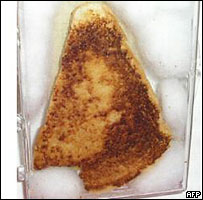[This article was first published on Robert Grant's stats blog » R, and kindly contributed to R-bloggers]. (You can report issue about the content on this page here)
Want to share your content on R-bloggers? click here if you have a blog, or here if you don't.

Recently, I was looking at some published research, and I became concerned that it looked strange. Firstly, it had results wildly different to other similar studies, and more exciting / publishable ones. So, I was looking through the original paper to try and work out what was going on. Something caught my eye in a table of means and standard deviations. All were given to one decimal place, and, as a fascinating article in Significance last year pointed out, you’d expect the digits 0-9 to appear with nearly the same frequency in the decimal points. Any imbalance should be no more than you’d expect by chance. But these ones looked odd. There seemed to be a lot of one digit, and quite a run of them all together in the table. I felt I needed to see just how unlikely it was that this could arise by sheer chance. If it was very unlikely, then  perhaps it would be time to start telling others that there might be something wrong here. In other words, I wanted to work out a p-value.The more you look, the more you see

As it happened, my fears were not backed up by the stats, so what I’ve described here is quite heavily disguised to protect the innocent from any mud sticking. But the process of investigating was quite interesting, so I thought I’d share it. I reached for R because I wanted to do something quite bespoke, and regular readers will not be surprised to hear I used a simulation approach. There probably is some weird test out there with asymptotic Z statistic, named after some long-dead guru of decimal points, but I could do the job more quickly and transparently with simulation.

```iter<-100000
# Data:
# decimal points in means

dp<-c(7,2,7,6,4,7,7,8,0,2,7,7,7,1,7,7,6,0,2,3,8,9,2,3,8,1,3,0,5,7,2,0,6,0,9,3,7,9,7,9,8,0,4,0,8,1,8,4)

# decimal points in standard deviations
dps<-c(7,7,1,7,3,8,6,4,2,8,8,2,5,9,4,1,0,0,8,6,7,3,0,8,6,5,1,8,1,4,2,9,1,0,5,6,4,3,3,6,3,4,9,3,2,1,6,9)

# Euclidean distance function - given 48 numbers we expect 4.8 of each digit
euc<-function(xx) {
sqrt(sum((xx-4.8)^2))
}

# Euclidean distance of dp
euc.dp<-euc(table(dp))
# simulate for multinominal distn under H0: prob(dp=x)=0.1 for all 0<=x<=9
sim<-rmultinom(iter,size=48,prob=rep(0.1,10))
euc.sim<-apply(sim,MARGIN=2,FUN=euc)
# this is the p-value:
sum(euc.sim>euc.dp)/iter # p=0.04

# Euclidean distance of dps
euc.dps<-euc(table(dps))
# this is the p-value:
sum(euc.sim<euc.dps)/iter # p=0.98
```

So, that didn’t look very conclusive; p=0.04 is borderline in my book. But that calculation didn’t take the runs into account. Another way of doing it would be to look at combinations of the ith digit and the (i+1)th.The more you see, the more you look

```# autocorrelation

euc2<-function(xx) {
sqrt(sum((table(xx[-48],xx[-1])-0.48)^2)) # there are 100 possible values now
}

euc.ac<-euc2(dp)
# simulate for multinominal distn under H0: prob(dp=x)=0.1 for all 0<=x<=9
sim<-matrix(floor(runif(48*iter,min=0,max=9.99999)),ncol=iter)
euc.sim.ac<-apply(sim,MARGIN=2,FUN=euc2)
# this is the p-value:
sum(euc.sim.ac>euc.ac)/iter # p=0.06

euc.acs<-euc2(dps)
# this is the p-value:
sum(euc.sim.ac>euc.acs)/iter # p=0.72
```

Even less convincing. Now for the really interesting stuff! Having looked at the same data in two different ways we should expect more than 5% type 1 error rate. I have committed the most basic form of multiple testing, running the same data through more than one pre-specified model. Worse than that, I chose the model by eyeballing the data, and I chose those data after scaning a whole published paper. Very questionable, the sort of thing Andrew Gelman described as researcher degrees of freedom. I had been eating up those degrees of freedom faster than a box of dates on Christmas day. With the lowest p-value being 0.04, pretty much any adjustment for multiplicity will mean there are no significant departures from a multinomial(0.1, 0.1, 0.1 …. 0.1) distribution. So, the verdict was not guilty.

Now, I’m not claiming that my heuristic choice of statistic is the best one to answer the question, or that I’ve tried the best models, or that the simulation equates to a Uniformly Most Powerful test. I’m just saying that it’s an interesting process to go through, from the fraud angle and also the multiplicity angle.